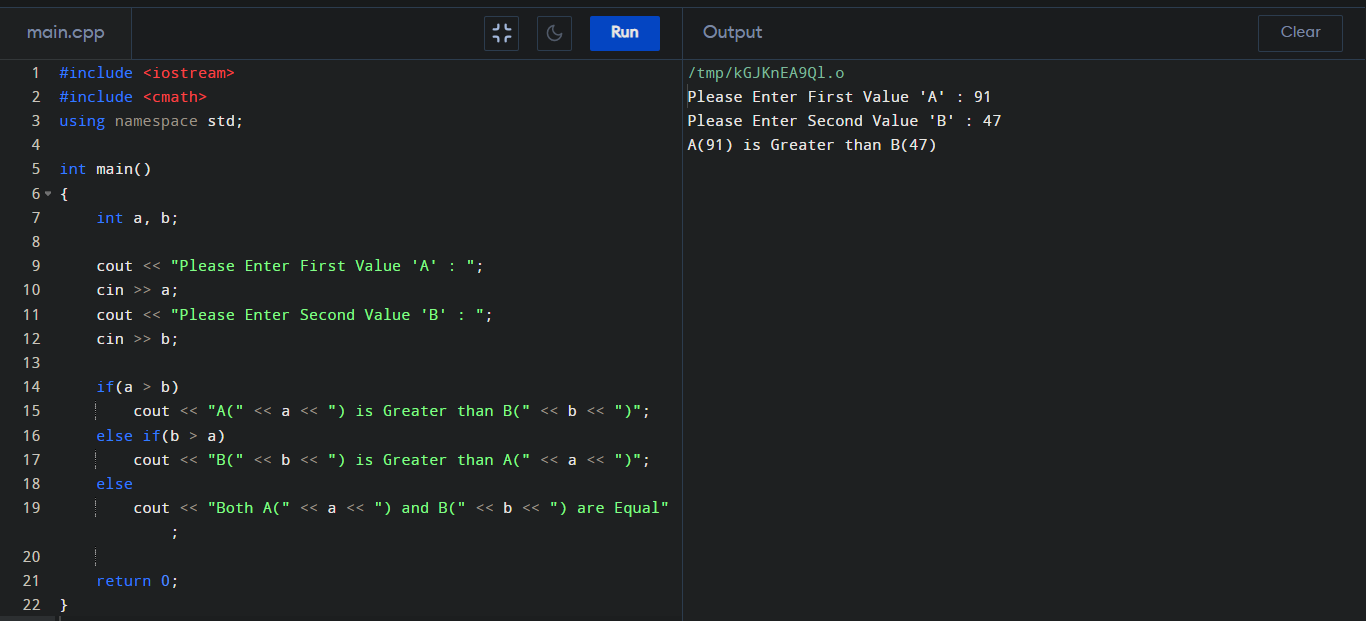C++ Program to find Greatest of Two Numbers

How to find the Greatest Number between two given Integer Number in C++? We can easily find the Greatest Value by using "if-else" Statement in C++.

Finding Greatest Number by C++ :

#include <iostream>
using namespace std;

int main()
{
int a, b;

cout << "Please Enter First Value 'A' : ";
cin >> a;
cout << "Please Enter Second Value 'B' : ";
cin >> b;

if(a > b)
cout << "A(" << a << ") is Greater than B(" << b << ")";
else if(b > a)
cout << "B(" << b << ") is Greater than A(" << a << ")";
else
cout << "Both A(" << a << ") and B(" << b << ") are Equal";

return 0;
}

Output :